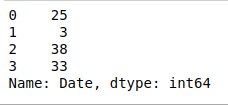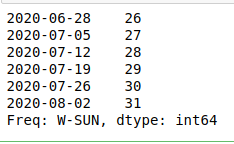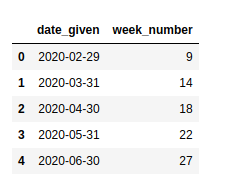# Extract week number from date in Pandas-Python

• Last Updated : 10 Jul, 2020

Many times, when working with some data containing dates we may need to extract the week number from a particular date. In Python, it can be easily done with the help of pandas.

Example 1:

 `# importing pandas as pd``import` `pandas as pd `` ` `# creating a dictionary containing a date``dict` `=` `{``'Date'``:[``"2015-06-17"``]}`` ` `# converting the dictionary to a dataframe``df ``=` `pd.DataFrame.from_dict(``dict``)`` ` `# converting the date to the required format``df[``'Date'``] ``=` `pd.to_datetime(df[``'Date'``], errors ``=``'coerce'``)``df.astype(``'int64'``).dtypes`` ` `# extracting the week from the date``weekNumber ``=` `df[``'Date'``].dt.week`` ` `print``(weekNumber)`

Output:

```0    25
Name: Date, dtype: int64```

Example 2: We can also do the same for multiple dates by adding more dates in the ‘Date’ object.

 `# importing pandas as pd``import` `pandas as pd `` ` `# creating a dictionary containing a date``dict` `=` `{``'Date'``:[``"2020-06-17"``, ``"2020-01-14"``, ``                ``"2020-09-20"``, ``"2020-08-15"``]}`` ` `# converting the dictionary to a ``# dataframe``df ``=` `pd.DataFrame.from_dict(``dict``)`` ` `# converting the date to the required ``# format``df[``'Date'``] ``=` `pd.to_datetime(df[``'Date'``],``                            ``errors ``=``'coerce'``)``df.astype(``'int64'``).dtypes`` ` `# extracting the week from the date``weekNumber ``=` `df[``'Date'``].dt.week`` ` `print``(weekNumber)`

Output:Example 3: Extracting week number from dates for multiple dates using date_range() and to_series().

• pandas.data_range(): It generates all the dates from the start to end date

Syntax:

pandas.date_range(start, end, periods, freq, tz, normalize, name, closed)

• pandas.to_series(): It creates a Series with both index and values equal to the index keys.

Syntax:

`Index.to_series(self, index, name)`

 `# importing pandas as pd``import` `pandas as pd `` ` `# generating all dates in given range``# with increment by days``allDates ``=` `pd.date_range(``'2020-06-27'``, ``'2020-08-03'``, freq ``=``'W'``)`` ` `# converting dates to series``series ``=` `allDates.to_series()`` ` `series.dt.week`

Output:Example 4: In this example, we’ll be using pandas.Series() to generate dates and use a different way to convert the series to the dataframe.

pandas.Series(): Used to create a one-dimensional ndarray with axis labels.
Syntax:

`pandas.Series(data, index, dtype, name, copy, fastpath)`

 `# importing pandas as pd``import` `pandas as pd `` ` `# generating the series``dates ``=` `pd.Series(pd.date_range(``'2020-2-10'``,``                                ``periods ``=` `5``,``                                ``freq ``=``'M'``))`` ` `# converting to dataframe``df ``=` `pd.DataFrame({``'date_given'``: dates})`` ` `# extracting the week number``df[``'week_number'``] ``=` `df[``'date_given'``].dt.week`` ` `df`

Output:My Personal Notes arrow_drop_up